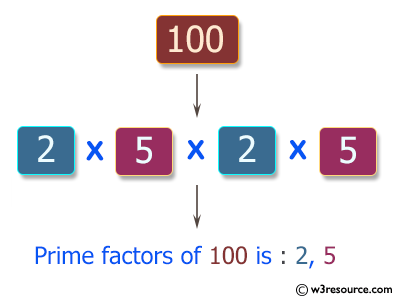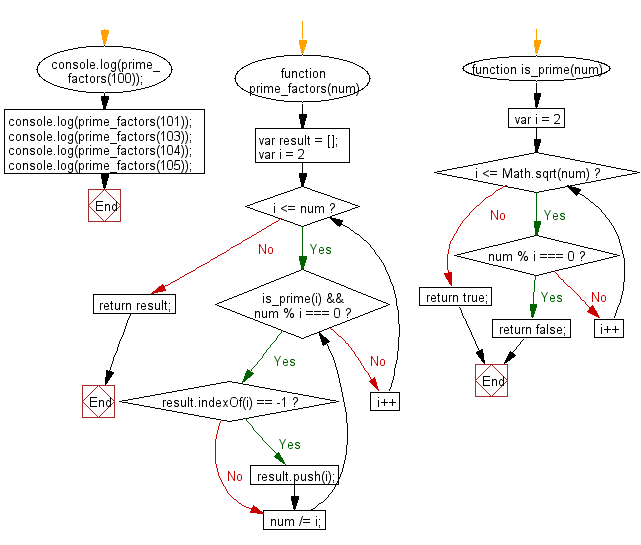# JavaScript: Find all distinct prime factors of a given integer

## JavaScript Basic: Exercise-132 with Solution

Write a JavaScript program to find all distinct prime factors of a given integer

Pictorial Presentation:Sample Solution:

HTML Code:

``````<!DOCTYPE html>
<html>
<meta charset="utf-8">
<meta name="viewport" content="width=device-width">
<title>Find all distinct prime factors of a given integer</title>
<body>

</body>
</html>
```
```

JavaScript Code:

``````function prime_factors(num) {
function is_prime(num) {
for (let i = 2; i <= Math.sqrt(num); i++)
{
if (num % i === 0) return false;
}
return true;
}
const result = [];
for (let i = 2; i <= num; i++)
{
while (is_prime(i) && num % i === 0)
{
if (!result.includes(i)) result.push(i);
num /= i;
}
}
return result;
}
console.log(prime_factors(100));
console.log(prime_factors(101));
console.log(prime_factors(103));
console.log(prime_factors(104));
console.log(prime_factors(105));
``````

Sample Output:

```[2,5]


[2,13]
[3,5,7]
```

Flowchart:ES6 Version:

``````function prime_factors(num) {
function is_prime(num) {
for (let i = 2; i <= Math.sqrt(num); i++)
{
if (num % i === 0) return false;
}
return true;
}
const result = [];
for (let i = 2; i <= num; i++)
{
while (is_prime(i) && num % i === 0)
{
if (!result.includes(i)) result.push(i);
num /= i;
}
}
return result;
}
console.log(prime_factors(100));
console.log(prime_factors(101));
console.log(prime_factors(103));
console.log(prime_factors(104));
console.log(prime_factors(105));
``````

Live Demo:

See the Pen javascript-basic-exercise-132 by w3resource (@w3resource) on CodePen.

Improve this sample solution and post your code through Disqus

What is the difficulty level of this exercise?

Test your Programming skills with w3resource's quiz.

﻿

## JavaScript: Tips of the Day

Checks if a string is an anagram of another string (case-insensitive, ignores spaces, punctuation and special characters)

Example:

```const isAnagram = (str1, str2) => {
const normalize = str =>
str
.toLowerCase()
.replace(/[^a-z0-9]/gi, '')
.split('')
.sort()
.join('');
return normalize(str1) === normalize(str2);
};
console.log(isAnagram('iceman', 'cinema')); // true
```

Output:

```true
```